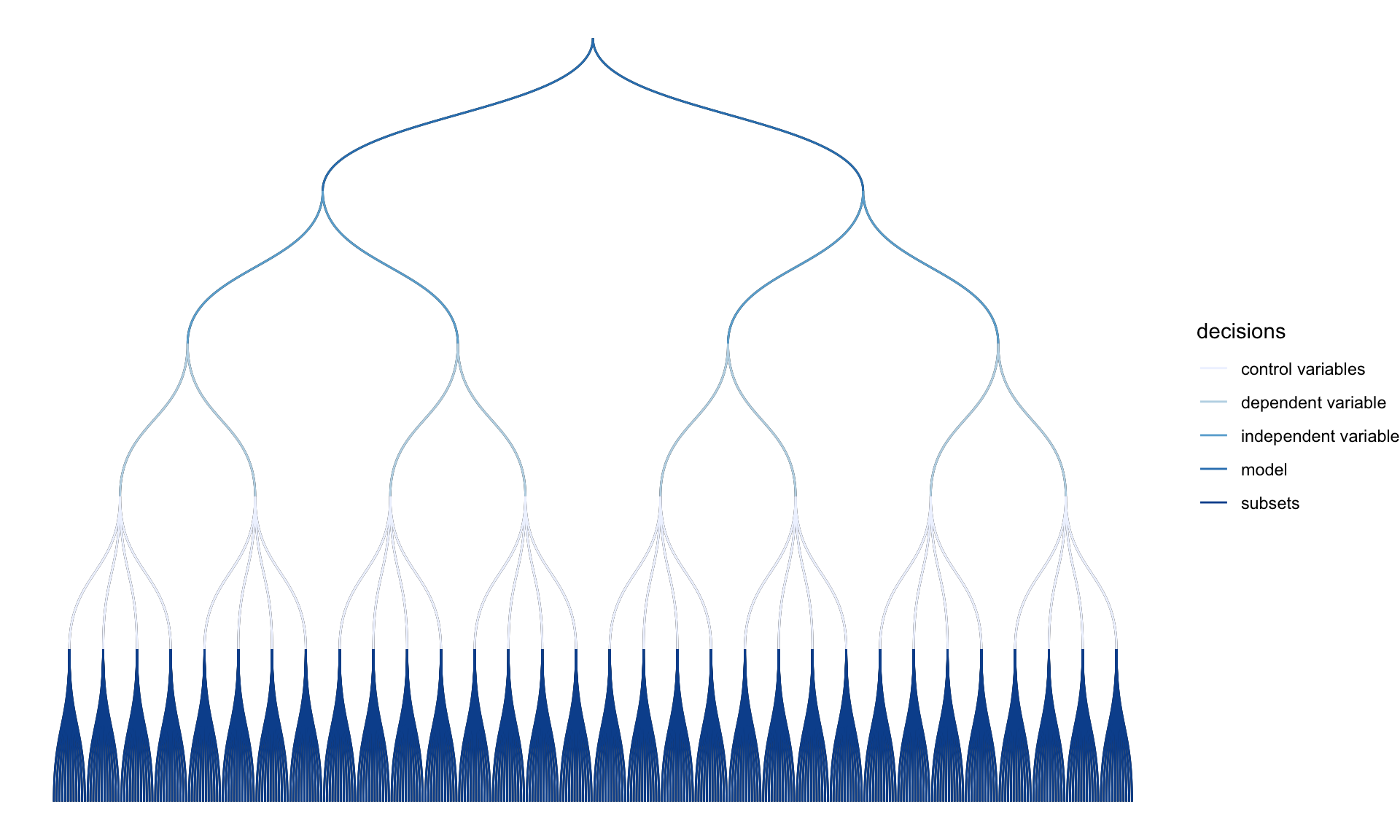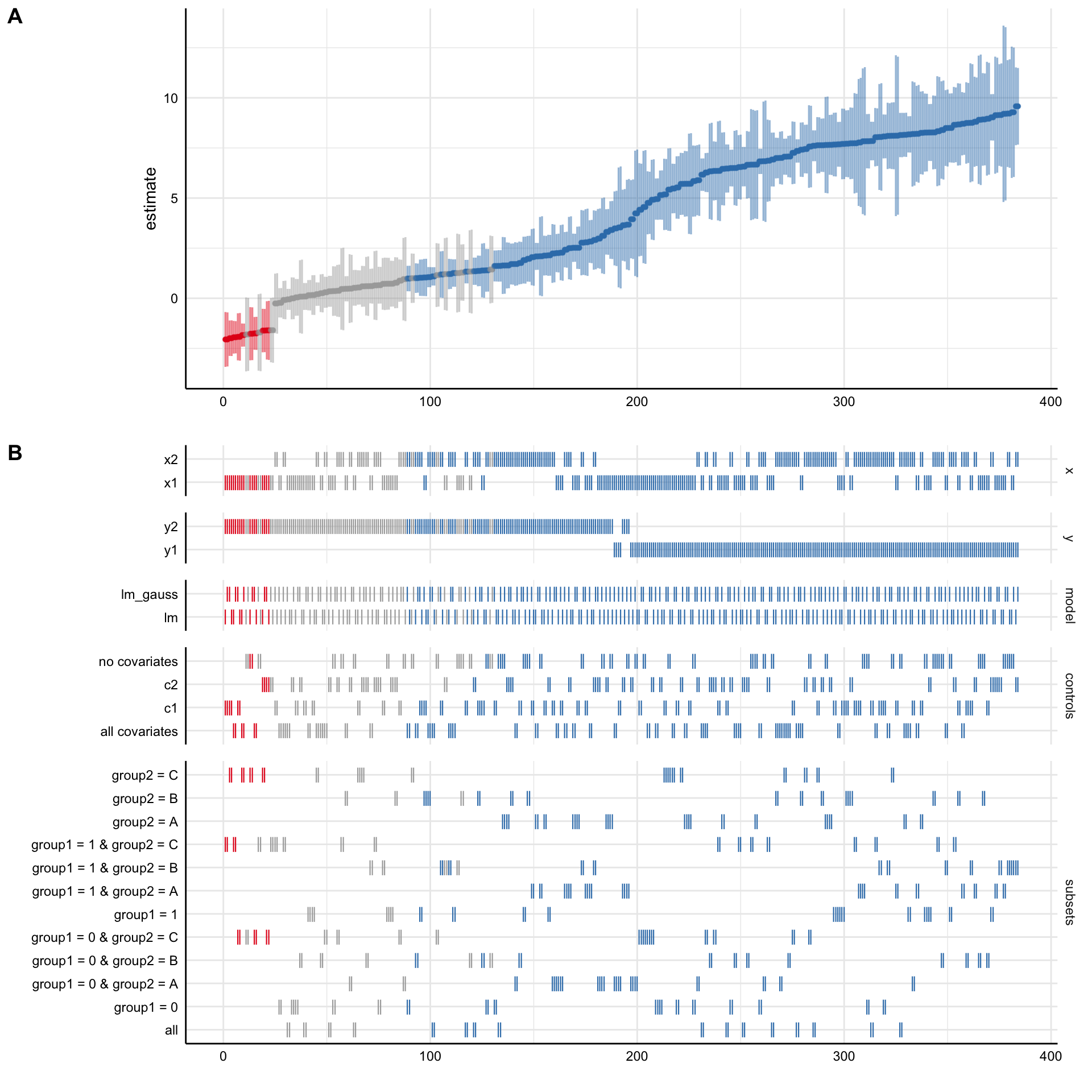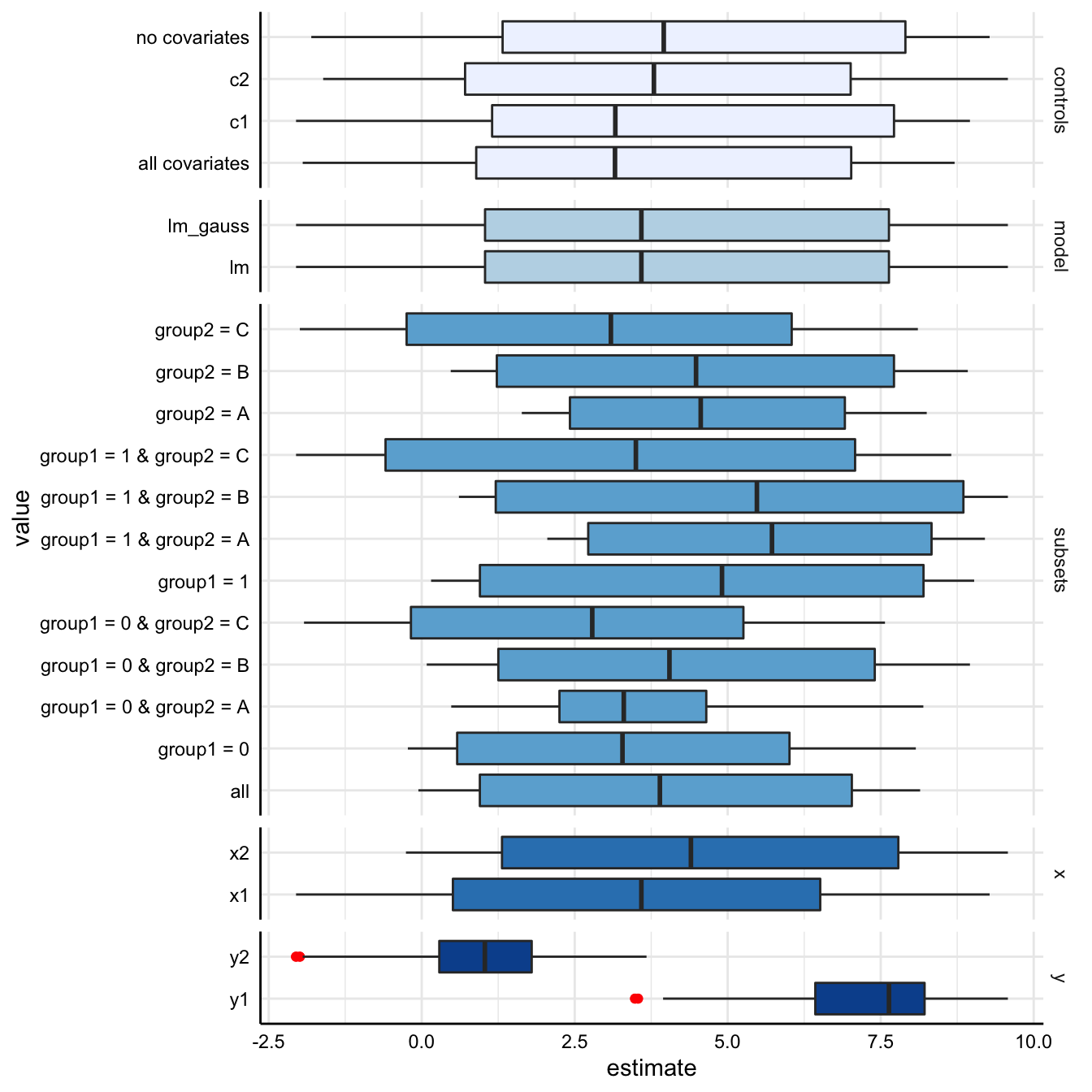# An introduction to specr

The following vignette is a more comprehensive version of the example on the homepage and exemplifies how to use the major functions of this package. Although using specr strictly speaking does not require any other package, we recommend to also load the tidyverse as it provides valuable functions for data wrangling and adapting outputs from specr functions. Furthermore, the tidyverse includes ggplot2 which can be used to modify the layout of the plots.

# Load libraries
library(specr)
library(tidyverse)

## 1. Check the data

In order to understand what type of analytical choices exists, you need to understand your data set. In a first step, you should hence investigate your data closely.

# We have a look at the simulated data set that is included in the package
#>         x1         x2         c1        c2        y1         y2 group1 group2
#> 1 1.533913  1.3697122  0.5424902 3.8924435 23.500543 10.4269278      0      A
#> 2 1.680639  1.5163745 -1.2415868 3.3377268 17.017955  0.5733467      1      C
#> 3 1.223941 -0.2381044  0.1405891 0.8911959 -3.678272  4.2303190      0      B
#> 4 1.765276  0.9524049  4.0397943 1.8567454 21.668684 14.8865252      1      B
#> 5 1.907134  0.6282816  3.1002518 5.5840574 32.713106 20.5251920      0      A
#> 6 1.710695  1.1898467  0.4648824 4.0239483 20.422171  4.3471236      1      C

# Summary of the data set
summary(example_data)
#>        x1               x2                c1               c2
#>  Min.   :-1.185   Min.   :-1.6037   Min.   :-4.093   Min.   :-3.780
#>  1st Qu.: 1.823   1st Qu.: 0.5248   1st Qu.: 1.242   1st Qu.: 1.282
#>  Median : 2.479   Median : 1.2740   Median : 2.513   Median : 2.775
#>  Mean   : 2.455   Mean   : 1.2999   Mean   : 2.499   Mean   : 2.690
#>  3rd Qu.: 3.192   3rd Qu.: 2.1049   3rd Qu.: 3.897   3rd Qu.: 4.064
#>  Max.   : 5.576   Max.   : 4.5741   Max.   : 7.994   Max.   : 9.001
#>        y1               y2              group1    group2
#>  Min.   :-22.93   Min.   :-22.148   Min.   :0.0   A:144
#>  1st Qu.: 12.50   1st Qu.:  3.944   1st Qu.:0.0   B:162
#>  Median : 21.69   Median : 11.753   Median :0.5   C:194
#>  Mean   : 23.89   Mean   : 11.200   Mean   :0.5
#>  3rd Qu.: 34.04   3rd Qu.: 17.966   3rd Qu.:1.0
#>  Max.   : 94.13   Max.   : 46.341   Max.   :1.0

There are several numeric variables. In this example, we assume that x represents independent variables, y represents dependent variables, c represents control variables, and group denotes potential grouping variables that can be used for subsetting the data.

## 2. Define analytical choices

The next steps involves identifying possible analytical choices. This step involves an in-depth understanding of the research question and the model(s) that will be specified. In this case, we assume simply that x should be positively correlated with y. We can use the additional function setup_specs() to check how different analytical decisions create varying factorial designs.

setup_specs(y = c("y1"),               # We choose only one dependent variale
x = c("x1", "x2"),         # We are not sure which independent variable is better
model = c("lm"),           # We only estimate one type of model (linear model)
controls = c("c1", "c2"))  # We include two control variable
#> # A tibble: 8 x 4
#>   x     y     model controls
#>   <chr> <chr> <chr> <chr>
#> 1 x1    y1    lm    c1 + c2
#> 2 x2    y1    lm    c1 + c2
#> 3 x1    y1    lm    c1
#> 4 x2    y1    lm    c1
#> 5 x1    y1    lm    c2
#> 6 x2    y1    lm    c2
#> 7 x1    y1    lm    no covariates
#> 8 x2    y1    lm    no covariates

The resulting data frame creates eight different specifications. setup_specs() can be used to check and understand how different analytical choices create specific combinations. Yet, this step is not mandatory. This helper function is used within the next function run_specs() that actually runs the specification analysis.

## 3. Define final analytical choices and run the analysis

The main function of the package is run_specs(). We need to include our analytical choices as arguments (as they will be passed to setup_specs().) Additionally, we need to provide the data and, if needed, we can also specify subsets that should be evaluated.

One type of analytical choice that could additionally affect the results refers to the type of model that is estimated. The function runs traditional linear regression models by default (i.e. when model = "lm" is provided as argument). However, customized model functions can be passed to the function, too. The only requirement is that the customized function has formula and data as argument names. The self-made function needs to be a model estimation function, that takes the formula and estimates the model based on the data. In run_specs(), the resulting object is passed to broom::tidy(), which can deal with a variety of model classes.

# specific model fitting function
lm_gauss <- function(formula, data) {
glm(formula = formula,
data = data,
}

# Run specification curve analysis
results <- run_specs(df = example_data,
y = c("y1", "y2"),
x = c("x1", "x2"),
model = c("lm", "lm_gauss"),
controls = c("c1", "c2"),
subsets = list(group1 = unique(example_data$group1), group2 = unique(example_data$group2)))

# Check
results
#> # A tibble: 384 x 12
#>    x     y     model controls estimate std.error statistic  p.value conf.low
#>    <chr> <chr> <chr> <chr>       <dbl>     <dbl>     <dbl>    <dbl>    <dbl>
#>  1 x1    y1    lm    c1 + c2     4.95      0.525     9.43  3.11e-18    3.92
#>  2 x2    y1    lm    c1 + c2     6.83      0.321    21.3   1.20e-57    6.20
#>  3 x1    y2    lm    c1 + c2    -0.227     0.373    -0.607 5.44e- 1   -0.961
#>  4 x2    y2    lm    c1 + c2     0.985     0.324     3.04  2.62e- 3    0.347
#>  5 x1    y1    lm_g… c1 + c2     4.95      0.525     9.43  3.11e-18    3.92
#>  6 x2    y1    lm_g… c1 + c2     6.83      0.321    21.3   1.20e-57    6.20
#>  7 x1    y2    lm_g… c1 + c2    -0.227     0.373    -0.607 5.44e- 1   -0.958
#>  8 x2    y2    lm_g… c1 + c2     0.985     0.324     3.04  2.62e- 3    0.350
#>  9 x1    y1    lm    c1          5.53      0.794     6.97  2.95e-11    3.96
#> 10 x2    y1    lm    c1          8.07      0.557    14.5   6.90e-35    6.98
#> # … with 374 more rows, and 3 more variables: conf.high <dbl>, obs <int>,
#> #   subsets <chr>

The resulting data frame includes relevant statistics for each of the estimated models.

We can now plot a simple decision tree to understand how our analytical choices lead to a large number of specifications.

plot_decisiontree(results,
legend = TRUE)## 4. Investigate the specification curve

The package includes a simple function summarise_specs() that allows to get a first summary of the results.

# basic summary of the entire specification curve
summarise_specs(results)
#> # A tibble: 1 x 7
#>   median   mad   min   max   q25   q75   obs
#>    <dbl> <dbl> <dbl> <dbl> <dbl> <dbl> <dbl>
#> 1   3.59  4.56 -2.05  9.58  1.03  7.63   123

# summary by specific groups and  statistics
summarise_specs(results,                         # result data frame
x, y,                            # grouping variables
stats = lst(median, min, max))   # specific functions
#> # A tibble: 4 x 6
#> # Groups:   x 
#>   x     y     median    min   max   obs
#>   <chr> <chr>  <dbl>  <dbl> <dbl> <dbl>
#> 1 x1    y1     6.52   3.49   9.28   123
#> 2 x1    y2     0.498 -2.05   3.67   123
#> 3 x2    y1     7.80   5.89   9.58   123
#> 4 x2    y2     1.29  -0.258  2.91   123

# summary of another statistic
summarise_specs(results,
subsets,
var = p.value)
#> # A tibble: 12 x 8
#>    subsets              median       mad      min    max      q25      q75   obs
#>    <chr>                 <dbl>     <dbl>    <dbl>  <dbl>    <dbl>    <dbl> <dbl>
#>  1 all                 1.84e-6   2.72e-6 5.08e-89 0.838  6.48e-40  6.31e-2   500
#>  2 group1 = 0          2.86e-5   4.24e-5 1.20e-57 0.994  2.33e-18  2.59e-1   250
#>  3 group1 = 0 & gr…    1.07e-3   1.59e-3 6.98e-16 0.577  2.35e- 5  4.95e-3    72
#>  4 group1 = 0 & gr…    7.86e-4   1.17e-3 2.53e-21 0.930  2.43e- 9  1.23e-1    76
#>  5 group1 = 0 & gr…    1.03e-3   1.53e-3 2.05e-22 0.572  2.37e- 8  6.66e-2   102
#>  6 group1 = 1          2.51e-5   3.71e-5 6.96e-46 0.749  3.52e-19  7.36e-2   250
#>  7 group1 = 1 & gr…    7.83e-5   1.16e-4 9.73e-17 0.0395 7.35e- 7  1.58e-3    72
#>  8 group1 = 1 & gr…    9.59e-5   1.42e-4 1.51e-15 0.277  1.32e- 9  7.74e-2    86
#>  9 group1 = 1 & gr…    7.58e-6   1.12e-5 3.86e-18 0.862  1.75e- 8  1.78e-1    92
#> 10 group2 = A          3.28e-8   4.86e-8 5.64e-30 0.0245 9.45e-11  9.52e-6   144
#> 11 group2 = B          2.87e-5   4.25e-5 2.05e-28 0.231  1.13e-15  8.32e-3   162
#> 12 group2 = C          3.03e-7   4.49e-7 2.45e-35 0.487  1.07e-15  3.48e-2   194

The output contains summary statistics such as the median, the median absolute deviation, … as well as the number of observations that were used for each model. Bear in mind that due to subsetting or missing data, sample sizes can vary considerably which, in turn, affects the results (e.g., the p-value).

However, in order to grasp how the different analytical choices affect the outcome of interest (in this case, the estimate refers to the unstandardized regression coefficient b), it is reasonable to plot a specification curve. The function plot_specs() to produces the typical visualization of the specification curve and how the analytical choices affected the obtained results.

# Plot specification curve analysis
plot_specs(results)The resulting plot includes the ranked specification curve (A) and an overview about how the different analytical choices affect the estimate of interest (B). Red represents negative and significant effects (based on the chosen significance level, by default $$\alpha = .05$$). Blue represents positive and significant effects. Grey refers to non-significant effects.

Sometimes, it can be useful to check the influence of specific choices on the estimate of interest more precisely. We can use the function plot_summary() to produce respective boxplots.

plot_summary(results)We can see that the dependent variable (y) produces the largest differences in the obtained estimates.

## 5. Decompose the variance in the specification curve

Finally, we can estimate how much variance in the specification curve is related to which analytical decisions. Therefore, we have to estimate a basic multilevel model without predictors and the analytical decisions as random effects (interactions could be included too). We then use the function icc_specs() to calculate a respective table or plot_variance() to visualize the distribution.

# Estimate multilevel model
library(lme4)
model <- lmer(estimate ~ 1 + (1|x)  + (1|y) + (1|controls) + (1|subsets), data = results)

# Get intra-class correlation
icc_specs(model) %>%
mutate_if(is.numeric, round, 2)
#>        grp  vcov  icc percent
#> 1  subsets  0.79 0.04    3.62
#> 2 controls  0.05 0.00    0.23
#> 3        y 19.74 0.90   89.83
#> 4        x  0.43 0.02    1.95
#> 5 Residual  0.96 0.04    4.38

# Plot decomposition
plot_variance(model)